Generating Norms for a Test

The following syntax files scores the subtests of depression (sdepress), anxious (sanxious), hurtself (shurdetl), and schizophrenia (sschiz).

Scoring Subtests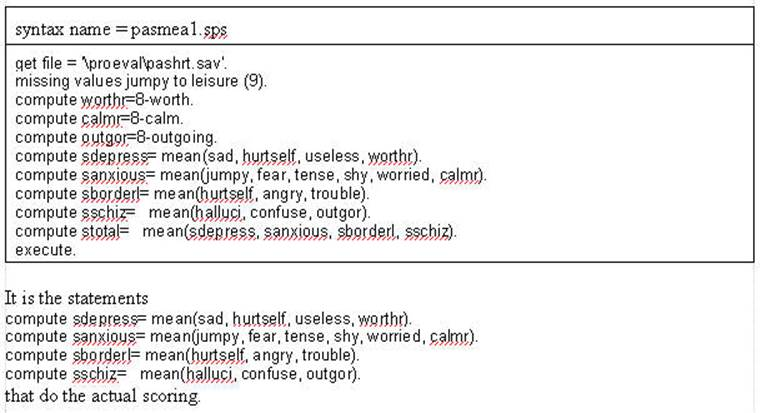Obtaining Means and Standard Deviations Needed for Norms

The following analysis assumes that the above syntax file has be run and that there is a diagnosis field where the person with the diagnosis field (DIAGN) contains: (1) anxious = 1, (2) depression = 2, (3) borderline = 3 and (4) schizophrenia = 4. Further, it is assumed that the diagnosis field (DIAGN) has been sorted in ascending order.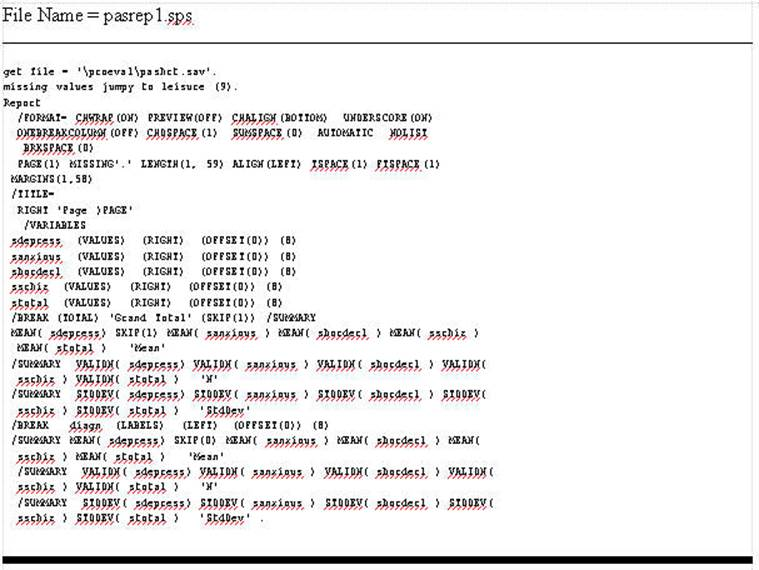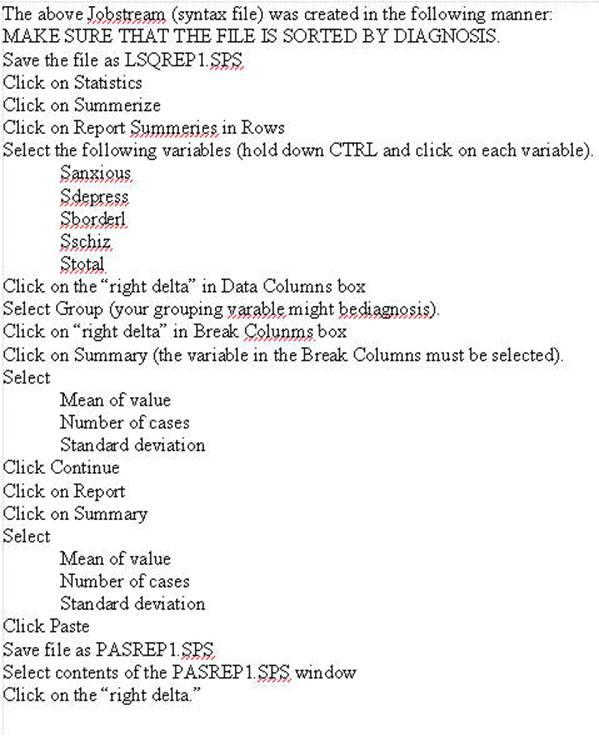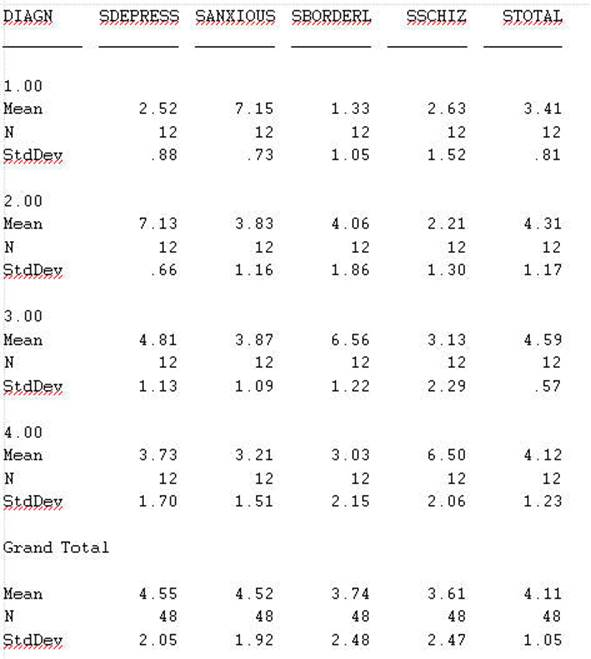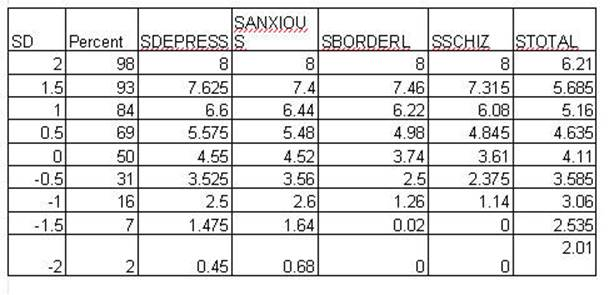The above chart is created in the following manner. The percentiles are taken from a normal curve function (found in most statistical text books) at the various statard deviations from the mean. For example, at the mean is the 50th percentile. The distance of 1 standard deviation from the mean contains 34.134 percent of the sample. Consequently, one standard deviation above the mean would contain 84 percent of the sampele (50 + 34). Further, one standard deviation below the mean would contain 16 percent of the sample (50 - 34).

The numbers are filled in the cells in the following manner. The means are filled in at 0 standard deviations from the mean. A 1 standard deviation above the mean the standard deviation of the sample score for that variable is added to the mean. For example, for the variable SDEPRESS the standard deviation of 2.05 is added to the mean (4.55) resulting in 6.6. When the standard deviation is subtracted from 4.55 (the mean) the result is 2.5. For the same variable 1/2 standard deviation is 1.025 and when that is added to the mean the result is 5.575. The remainder of the table is completed in the same manner.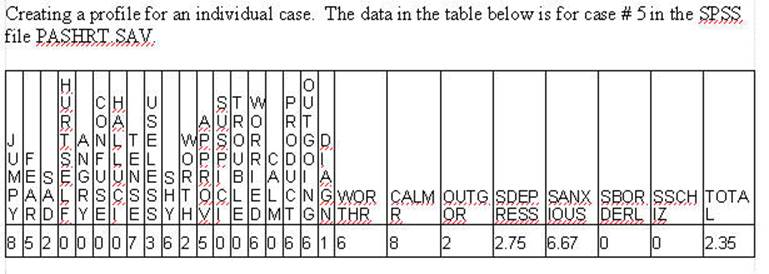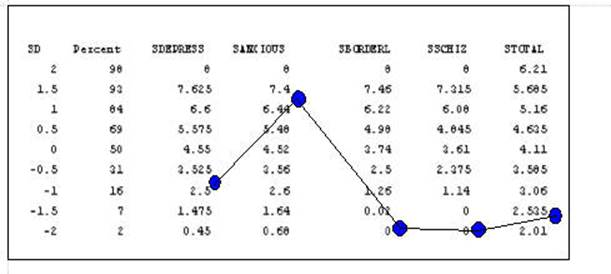The subtests can be plotted in most wordprocessing programs using the Draw command as presented in the next figure.

[NOTE:  The table can be created in a wordprocessing program such as Word using Tables.]

(Standard Scores)